Journal of World Economic Research
Volume 4, Issue 5-1, September 2015, Pages: 33-39

The Causality between Economic Growth and the Environmental Quality: Implementing the Hsiao Causality Test Using Regression Model and Artificial Neural Network (ANN)

Morteza Hasanshahi1, Majid Zare2, *

1Department of Economics, College of Economics, Arsanjan Branch, Islamic Azad University (IAU), Arsanjan, Iran

2Department of Agricultural Economics, Arsanjan Branch, Islamic Azad University (IAU), Arsanjan, Iran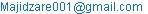(M. Zare)

Morteza Hasanshahi, Majid Zare. The Causality between Economic Growthand the Environmental Quality: Implementing the Hsiao Causality Test Using Regression Model and Artificial Neural Network (ANN). Journal of World Economic Research. Special Issue: The Globalization and Economic Structure Changes. Vol. 4, No. 5-1, 2015, pp. 33-39. doi: 10.11648/j.jwer.s.2015040501.15

Abstract: The aim of the present study was to determine the causality between economic growth and environmental quality. The variables under study were economic growth, government expenditure, openness degree of the economy, capital stock, population, average export of contaminants, and carbon dioxide (CO2) emissions during the time period from 1977 to 2010 for the Iranian economy. The results suggested that there is a two-way relationship between economic growth and environmental quality. Besides, economic growth was found to have the greatest impact on air pollution. It was also noted that the artificial neural network (ANN) produces better results (estimates) in most cases than the regression model.

Keywords: Economic Growth, CO2 Emission; Environmental Quality (Air Pollution), Artificial Neural Network (ANN)

1. Introduction

During the last two decades, the relationship between the communities’ development level and the achievement of environmental standards or environmental considerations has received great attention by researchers. This has been also focused upon in economics using a special approach. One of the issues that have been widely researched is the relationship between the communities’ income level and environmental destruction level. A line of research in this field shows that two intellectual approaches have been taken during the recent decades that have ultimately led to a third approach. The first approach, known as Antigrowth Theory, states that the economic growth necessarily leads to the environmental destruction as growth means to inject much raw materials and energy into the economic system and thus generating more wastes. Therefore, the economic growth not only endangers scare resources but also presents risks to the environment by producing waste materials behind the capacity of the natural environments. In addition, there is a second approach known as Economic Growth Theory. This theory assumes that growth is a means to provide the needed capital for the protection of the environment. Therefore, economic growth can improve the quality of the environment rather than threatening it. As economic growth generates more products and services, there will be more motivation to support the environment and it gains significance in political programs. Besides, various methods such as resources substitution, technological innovations, and changes in the demand patterns when changing relative prices to protect the environment will emerge. The conflict between the two approaches and the fundamental differences between their underlying assumptions along with various experimental evidences resulted in the emergence of a third approach in the 1990s. According to this approach, the relationship between the economic growth and the environment quality, positive or negative, in the development path of each country is not a stable relationship. In fact, such a relationship will be reversed over time as income reaches a given level. Such a U-shaped reverse relationship is known in the economic growth and environment literature as Environmental Kuznets Curve (EKC) or Environmental Transmission Hypothesis (ETH). Many studies have been conducted in the recent years on the relationship between economic growth and pollution. Although most studies have pointed to the existence of a U-shaped relationship for some pollutants, there is no observation showing that EKC could be applied to all pollutants or different types of environmental problems. It should be also noted that much of the discussion has been limited to developed counties. Besides, in most cases, studies have sought to find an answer to the question whether a U-shaped relationship applies to all pollutants or not or if such a relationship can be found in developing countries as is the case with the developed nations. The other issue of interest is at which income level EKC would be reversed and how it is possible to apply experiences from cross-county studies to a given country in order to estimate the environmental development path. Besides, it would be interesting to determine the role played by environmental policies in the relationship between the economic growth and the environment as well as in reducing environmental cost for the purpose of achieving sustainable development. One of the main problems faced in such studies is the lack of sufficient research on theoretical resources and determining factors affecting the relationship between the economic growth and the environment. As such, the present study tries to develop a general framework to evaluate the relationship between economic and the environmental destruction and to determine the main factors affecting the relationship between the economic growth and the environment using artificial neural network and regression. The findings of this study has some policy implications for developing countries which are at initial stages of development and are going to grapple with environmental problems.

1.1. Theoretical Framework of the Study

The Hsiao causality test which is devised based on the Environmental Kuznets Curve (EKC) was employed in this study. The reason is that the results of Granger Causality Standard Test are very sensitive to the selection of lag length .To deal with this problem, Hsiao (1981) has developed a systematic autoregressive method for choosing optimal lag length for each variable in an equation. Both methods are based on the Environmental Kuznets Curve (EKC).

1.2. Environmental Kuznets Curve (EKC)

Simon Kuznets (1955), in a study entitled "Economic growth and income inequality" introduced an inverted U-curve known as Kuznets Curve. According to him, the relationship between per capita income and income distribution is in the form of an inverted U-shaped patternin the course of economic development. According to this hypothesis, in the early stages of economic development; income distribution inequality will increase simultaneously with the increased per capita income and there will be a certain point after which income distribution inequality will decline gradually. In the 1990s when some evidence pointed to the existence of a U-shaped pattern between environmental destruction indices and per capita income, the Environmental Kuznets Curve (EKC) started to be used in environmental studies.

2. Literature Review

Grossman and Krueger (1993), found a U-shaped pattern between pollution and per capita income. In contrast, De Bruyn et al., (1998), concluded that such U-shaped pattern does not exist in the long run and it can only be seen in the early stages of economic development as there will be a certain point after which this pattern will turn into an N-shaped curve (suggesting that environmental damages will reappear at high economic growth levels). Similarly, Unruh and Moomaw (1998), using data from 1950-1992 demonstrated the nonexistence of the EKC hypothesis in 16 countries. Simultaneously, De Bruyn et al., (1998), conducted a study in four countries, namely Netherlands, UK, USA, and Germany for the time intervals between 1960 and 1993. They found no relationship between economic growth and emissions from CO2, NOx, and SO2. Agras and Chapman(1999), using a dynamic approach, confirmed the EKC hypothesis in 34 countries in 1971-1989 time period. Friedl and Getzner (2002), demonstrated an N-shaped relationship between economic growth and pollution. Murthy et al., (2006), using a multidimensional model for India in a 35 year time horizon, showed that CO2 emissions reduction would result in lower GDP and grater poverty. In addition, Jiang et al., (2008), Olusegun (2009), Lean and Smyth (2012), Iwata et al., (2009), Annicchiarico et al., (2009), conducted similar studies to estimate the Environmental Kuznets Curve.

3. Research Model and How It Is Estimated

The Hsiao causality test which posits economic growth as a function of the environmental quality was used in this study:Where, X shows pollution level and Y stands for economic growth. The above equations can be written as a causality equation in the form of an auto-regressive vectors model: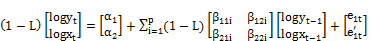(1)

Or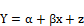Where, β =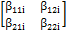, α =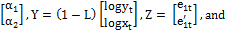X =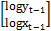.

Alternatively, environmental quality can be assumed as a function of economic growth. To implement the Hsiao test, it is needed to know the values of the Y,X optimal lags that are shown by m* and n*, respectively. The values of the final prediction error with Y optimal lag (FPE(m*)) and the final prediction error with Y , X optimal lags (FPE(n*,m*)) are estimated.

To calculate the lag length in the above model, the Granger test and the final prediction error are combined. In the first step, the lag length is determined for X (i = 1, …, m) and in the second step, the lag length is determined for Y (i = 1, …, n). to do so, a set of autoregressive models are estimated as follows so that in the first regression model, the variable dependent lag is initiated from one and another lag is added in the next regression and the same procedure will continue as much as possible.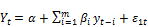(3)

In the next stage, the values of final prediction error (FPE) are calculated for each equation as follows: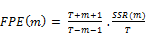(4)

Where, T stands for the sample size, SSR is the Sum of Squared Residuals, and m* is the optimal lag length (i.e. the lag that minimizes the FPE value). In the next stage, the following regression equation is used to determine X lags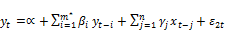(5)

Then the FPE values for each regression equation are calculated as follows:(6)

The optimal lag for X is a lag which minimizes the value of FPE. The Hsiao causality test make a comparison of FPE(m*) and FPE(m*,n*). If FPE(m*) <FPE(m*,n*), X will not be the cause of Y. On the other hand, If FPE(m*) >FPE(m*,n*), X will be considered as the cause of Y. in the Hsiao test, all variables are needed to be reliable. Otherwise, their reliability differences will be used.

4. Data Analysis and Empirical Results

To separate the impacts of environmental variables, the important variables affecting economic growth were included into the model as follows:

CO2 is the per capita CO2 emissions (tons), RGDP represents gross domestic product (billion dollars), G shows government expenditures, T is the capital stock (million dollars), OPEN is the openness degree of the economy (the ratio of the sum of exports and imports to the GDP), APO is average export of contaminants (tons), and POP is populations (1000 persons).

5. Variables Reliability Analysis

The reliability analysis of variables under study shown in Table 1 suggests that some research variables such as economic growth, CO2, openness degree of the economy, capital stock, and government expenditure are not reliable while population and the average export of contaminants are reliable. As a result, VAR must be used.

Table 1. Results of Variables Reliability Analysis (Philip - Prawn Test).

 Variables (log) Philips Prawn statistics Critical values Results 1% 5% RGDP first-order difference 3.14- 3.67- 2.96- Reliable CO2 first-order difference 3.84- 3.67- 2.96- Reliable APO 5.01- 4.29- 3.56- Reliable OPEN first-order difference 5.02- 3.67- 2.96- Reliable T first-order difference 5.7- 4.41- 3.62- Reliable G first-order difference 3.89- 4.30- 3.57- Reliable POP 6.95- 3.67- 2.96- Reliable

6. Cointegration Test

The results of the cointegration test indicate that there is no relationship between paired variables.

Optimal Lag Estimation:

A. The optimal lag of the independent variables was determined through the following stages:

1. First the following modelwas calculated:

DL RGDPt = a + b1. DLRGDPt-1+ b2. DLRGDPt-2 + b3. DLRGDPt-3+ b4. DLRGDPt-4+ b5. DLCO2t-1+ et

Where, the independent variables are RGDPt with 4 lags and CO2 with different lags. As was the case with previous stage, SSR and FPE values were determined using the following equation: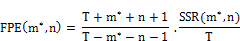2. In the second stage, the following model was fitted (m=2) and the FPE value was recalculated:

DL RGDPt = A + b1. DLRGDPt-1 +b2. DLRGDPt-2 + et

3. The same procedure was repeated for five lags (m=5) and SSR and FPE values were calculated. Then, FPE values were compared and the lag with the smallest FPE value was selected as the optimal lag of the dependent variable. As shown in Table 2, FPE in Lag 4 has the smallest value which equals 0.001968. As a result, it is the optimal lag which shows that the economic growth is influenced by its four last periods.

Table 2. Optimal lag with the dependent variable (RGDP) in the first stage .

 Number of lags SSR FPE(m) 1 0.091442 0.003443 2 0.056045 0.002283 3 0.049556 0.002160 4** 0.042166 0.196700 5 0.042006 0.002100

** The optimal lag minimizes the FPE value

B. The optimal lag of the independent variables was determined through the following stages: 1. First the following modelwas calculated:

DL RGDPt = a + b1. DLRGDPt-1+ b2. DLRGDPt-2 + b3. DLRGDPt-3+ b4. DLRGDPt-4+ b5. DLCO2t-1+ et

Where, the independent variables are RGDPt with 4 lags and CO2 with different lags. As was the case with previous stage, SSR and FPE values were determined using the following equation:Where, m* = 4, N is the lag for the independent variable (CO2) which is originally equal to 1.

2. Then, the model was estimated as follows:

DLGDP= a+b1. DLGDPt-1+ b2. DLGDPt-2+ b3. DLGDPt-3 + b4. DLGDPt-4+ b5. DLCO2t-1 + b6. DLCO2t-2+ et

It shows that CO2 was estimated with two lags and then SSR and FPE values were calculated.

3. The same procedure was repeated for four lags and each time, FPE values were calculated. Then, FPE values were compared and the lag with the smallest FPE value was selected as the optimal lag of the independent variables. For instance, the FPE for Co2in Lag 2 has the smallest value and equals 0.001746. This procedure was repeated for all independent variables (The results for other independent variables have been provided only for optimal lags). Table 3 shows the results related to all independent variables. In this table, the optimal lags for export of contaminants (DLAPO), government expenditure, capital stock (DLI), openness degree of the economy (DLOPEN), and population (DLPOP) are 5, 1, 5, 4, and 1, respectively. In this table, L stands for logarithm.

Table 3. Results of Hsiao Causality Test withr GDP and other variables in the second stage .

 Models Number of lags SSR FPE(m) DLRGDP =a.+ DLRGDP (4.1)+DLCO2(1.1)+et **2-(*4) 0.042137 0.002107 DLRGDP =a.+ DLRGDP (4.1)+DLCO2(2.1)+et 3-(4) 0.032562 0.001746 DLRGDP =a.+ DLRGDP (4.1)+DLCO2(3.1)+et 4-(4) 0.032509 0.001872 DLRGDP =a.+ DLRGDP (4.1) +DLCO2(4.1)+et **5-*(4) 0.032456 0.002010 DLRGDP =a.+ DLRGDP (4.1)+DLAPO(5.1)+et **1-(4) 0.012586 0.000839 DLRGDP =a.+ DLRGDP (4.1)+DLG(1.1)+et **5-*(4) 0.040385 0.002019 DLRGDP =a.+ DLRGDP (4.1)+DLI(5.1)+et *4-**(4) 0.024509 0.001614 DLRGDP =a.+ DLRGDP (4.1)+DLOPEN(4.1)+et **5-*(4) 0.025204 0.001560 DLRGDP =a.+ DLRGDP (4.1)+DLPOP(5.1)+et **2-(*4) 0.016904 0.001127

*The optimal lag for the dependent variable, ** Optimal lags for independent variables

Assuming CO2 and APO as the dependent variables, the above steps were repeated for the model under study as shown in Table 4:

Table 4. Optimal lag length with LCO2 in the first stage.

 Number of lags SSR FPE(m) 1** 0.0250134 0.0009574 2 0.0255770 0.001042 3 0.025029 0.001091 4 0.024977 0.001165 5 0.23874 0.001193

** Optimal lag

As shown in the above table, the optimal lag with CO2 as the dependent variable (dLCO2) is 1.

Table 5. Results of Hsiao Causality Test with RGDP as the dependent variable and other variables in the second stage .

 Models Number of lags SSR FPE(m) DLCO2=a.+DLCO2(1.1)+DLRGDP(4.1)+et **4-*(1) 0.017409 0.00087 DLCO2=a.+DLCO2(1.1)+DLRGDP (4.1)+et **1-*(1) 0.017097 0.00086 DLCO2=a.+DLCO2(1.1)+DLI(1.1)+et **1-*(1) 0.018129 0.00074 DLCO2=a.+DLCO2(1.1)+DLOPEN(1.1)+et **1-*(1) 0.025104 0.001023 DLCO2=a.+DLCO2(1.1)+DLPOP(1.1)+et **1-*(1) 0.024790 0.00101

*The optimal lag for the dependent variable, ** Optimal lags for independent variables findings)

As shown in Table 5, the optimal lag length for economic growth (LRGDP) and government expenditure (LG) equals 4 and its corresponding value for capital stock (LI), openness degree of the economy (DLOPEN), and population (LPOP) is 1.

Table 6. Optimal lag length with APO in the first stage.

 Number of lags SSR FPE(m) 1 1.725878 0.06575 2** 1.526797 0.06421 3 1.513103 0.06595 4 1.466198 0.06842 5 1.427549 0.07137

** Optimal lag

As shown in Table 6, when the export of contaminants is taken as the dependent variable (LAPO) is equal to 2.

Table 7. Results of Hsiao Causality Test with APO as the dependent variable and other variables in the second stage .

 Models Number of lags SSR FPE(m) DLCO2=a.+DLCO2(1.1)+DLPOP(1.1)+et **4-*(2) 0.068394 0.003938 DLCO2=a.+DLCO2(1.1)+DLPOP(1.1)+et **5-*(2) 0.114588 0.064173 DLCO2=a.+DLCO2(1.1)+DLPOP(1.1)+et **3-*(2) 0.118008 0.0559 DLCO2=a.+DLCO2(1.1)+DLPOP(1.1)+et **2-*(2) 0.756230 0.032291

*The optimal lag for the dependent variable; ** Optimal lags for independent variables (Resource: Research findings)

According to Table 7, the optimal lag for the economic growth, government expenditure, capital stock, and openness degree of economy are 4, 5, 3, and 2, respectively.

7. Model Estimation

This section presents the results of the Hsiao model (Model 1).

A. First, RGDP is taken as the dependent variable and then the independent variables are added into Model 1 separately and FPE(m*) and FPE(m*,n*) are calculated and compared. If FPE(m*,n*)<FPE(m*), changes in the independent variable are considered as the cause of the dependent variable changes. The last column in Table 8 presents the results of the Hsiao causality test.

Table 8. Summary of the results of the Hsiao test with LRGDP as the dependent variable.

 Independent variables m* FPE(m*) n* FPE(m*,n*) Results DLCO2 4 0.001967 2 0.001746 Co2 is the cause of RGDP DLG 4 0.001961 1 0.002019 G is not the cause of RGDP DLOPEN 4 0.001963 4 0.00156 OPEN is the cause of RGDP DLPOP 4 0.001966 5 0.001127 POP is the cause of RGDP DLI 4 0.00190 5 0.001614 I is the cause of RGDP DLAPO 4 0.001965 4 0.000904 PO is the cause of RGDP

As shown in the above table, since FPE(m*) > FPE(m*,n); it is concluded that CO2 emission, openness degree of economy, population, capital stock, and the export of contaminants affect economic growth.

B. In this stage, CO2 is taken as the dependent variable and the same procedure was repeated as did in the previous stage. The results are summarized in Table 9:

Table 9. Results of the Hsiao test with CO2 as the dependent variable.

 Independent variables m* FPE(m*) n* FPE(m*,n*) Results LRGDP 1 0.0009574 4 0.001746 RGDP is the cause of CO2. LI 1 0.0009574 1 0.002019 I is the cause of CO2. LOPEN 1 0.0009574 1 0.00156 OPEN is the cause of CO2. LPOP 1 0.0009574 1 0.001127 LPOP is not the cause of CO2. LG 1 0.0009574 4 0.001614 LG is the cause of CO2.

As evident in this table, economic growth, government expenditure, and investment will increase CO2 emissions. In contrast, openness degree of economy and population do not affect CO2 emissions.

Table 10. Results of the Hsiao test with LAPO as the dependent variable.

 Effective variables m* FPE(m*) n* FPE(m*,n*) Results LRGDP 2 0.06421 5 0.003938 RGDP is the cause of LAPO. LI 2 0.06241 3 0.0559 I is the cause of LAPO. LOPEN 2 0.0643 2 0.03529 OPEN is the cause of LAPO. LPOP 2 0.06621 2 0.06405 LPOP is not the cause of LAPO. LG 2 0.06431 5 0.06417 LG is the cause of LAPO.

Overall, the findings indicate that economic growth, openness degree of economy, government expenditure, and investment will increase CO2 emissions.

8. Data Analysis Using Artificial Neural Network (ANN)

To train and test the network in the ANN, the data are divided into two groups as typically done in conventional prediction methods. After training the network and stopping the learning process, the holdout sample is used to explore the network efficiency. In this study, approximately 90% of the data collected were used as the training sample and the remaining 10% of the data were used as the holdout sample. The number of neurons in the hidden layer of the network varied from 1-5 neurons. The model data and the ANN outputs are shown in tales 11-15. (Given the numerous results obtained from the ANN, only the results related to the economic growth as the dependent variable and those of air pollution as the independent variables are presented and compared with the results from the regression model).

Table 11. ANN summery statistics.

 Details Number Percentage Sample Training 30 88 Holdout 4 12 Valid 34 100 Total 34

Table 12. Network data.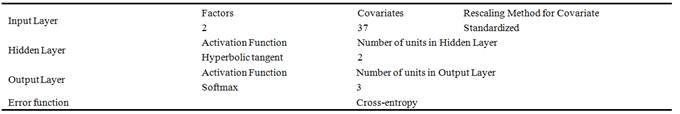Table 13. Model Summery (Economic growth = Dependent variable).

 Training Cross Entropy Error 12 Incorrect Predictions (%) 0.027 Stopping Rule Used Maximum number of epochs exceeded Holdout Incorrect Predictions (%) 24.5%

Table 14. Economic growth prediction using ANN.

 Sample Observed Prediction 10- 0 20- 11 30- 21 Correct percentage Training 11- 0 5 0 0 100 23- 12 0 46 0 100 34- 24 0 4 54 93 Overall Percentage 4.6% 46% 49% 96 Holdout 11- 0 0 0 0 0 23- 12 3 9 1 70 34- 24 1 5 22 79 Overall Percentage 10% 34% 56% 76

As shown in the above table, 96% of the predictions made by the ANN are correct while this amounts to 87% when using the regression model.

Table 15. Rating variables using ANN.

 CO2 (Dependent variable) RGDP (Dependent variable) Rating Standardized Sig. Sig. Variables Rating Standardized Sig. Sig. Variables 9 34% .020 CO2 t-1 8 11% .012 CO2 1 100% .06 RGDP 1 100% .11 APO 7 43% .026 G 5 44% .048 G 4 58% .035 T 3 50% .055 T 6 52% .031 OPEN 2 56% .062 OPEN 5 55% .033 POP 6 16% .018 POP 2 67% .040 APO 4 46% .051 RGDPt-1 10 18% .011 CO2t-2 7 15% .016 RGDPt-2 3 63% .038 RGDPt 9 09% .010 RGDPt-3 8 38% .023 G 10 05% .006 RGDPt-4

As evident in the above table, the average export of contaminants (APO) has the strongest impact (0.11) on the economic growth (RGDP). The value of the standardized significance coefficient was calculated as the value of significance coefficient divided by 0.11 which measures the impact of the variables on the dependent variables relative to APO. In addition, the economic growth was reported as the most important cause of air pollution.

9. Conclusions

The results of descriptive analysis of the data indicate that the per capita NOx emission of 15.4 kg in 2001 has risen to 24.9 kg in 2008. Besides, the per capita SO2 emission of 18.2 kg has increased to 22 kg in the same period. The same figures for CO2 and CO2 emissions are 46.85 vs. 7209.5 and 92.8 vs. 123.6 kg in the period under study. It was also noted that the per capita emissions of CH4 and N2O as greenhouse gases in 2008 are estimated to be 0.6 and 0.2, respectively.

Moreover, the results of the model estimation suggest:

Ÿ The increased emissions from CO2 and other contaminants (e.g. the construction of factories) will increase the economic growth.

Ÿ The economic growth will increase emissions from CO2 and other contaminants.

Ÿ The increased levels of capital stocks and government expenditure will result in an increase in CO2 emissions.

Ÿ The variations in the openness degree of the economy and the population will not affect CO2 emissions.

Ÿ The higher levels of capital stocks, the openness degree of the economy, and government expenditure will not increase contaminants.

Ÿ Population increase or decrease will not affect the emissions of contaminants.

References

1. Agras, J., Chapman, D., (1999). A dynamic approach to the Environmental Kuznets Curve hypothesis. Ecological Economics 28 (2), 267-277.
2. Annicchiarico, B., A.R., Bennato, and A. Costa (2009). Economic growth and carbon dioxide emissions in Italy, 1861 –2003, Department of Economics, University of Rome Tor Vergata, Online athttp://mra.ub.unimuenchen.de/12817/MPRApaper, No. 12871.
3. De Bruyn, S,M., van den Bergh, J.C.J.M., Opschoor, J.B., (1998). Economic Growth and Emissions: Reconsidering the Empirical Basis of Environmental Kuznets Curves, Ecological Economics 25, 161 – 175.
4. Friedl, B., and M. Getzner (2002). Environmental and growth in a small open economy: av EKC case-study for Austrian CO2 emission, Discussion paper of the college of business administration university of Klagenfurt, Austria.
5. Grossman, G.M. and A.B. Krueger (1993). "Environmental Impacts of a North American Free Trade Agreement" in P. Garer, ed., The U.s.-Mexico Free Trade Agreement. Cambridge, MA: MIT Press.
6. Iwata, H., K. Okada, and S. Samreth (2009). Empirical study on the environmental Kuznets Curve for CO2 in France: The roleof nuclear energy, Online at-http://mpra.ub.uni-muenchen.de/18997/MPRA paper No. 18997, posted 03.
7. Kuznets, S., (1955). economic growth and income inequaligy, American Economic Review 45, pp.1-28.
8. Jiang, Y., L. Tun, and Z. Juzhong (2008). Environment Kuznets Curves in the Peopele’s Republic of china: turning points and regional differences, available from:-http://mpra.ub.uni-muenchen.de/21252/.
9. Lean, H., and R. Smyth (2012). CO2 emission, electricity consumption and output in ASEAN
10. Murthy, S.N., M. panda, and K. Parick (2006). CO2 emissions reduction strategies and economic development of India, Indira Gandhi Institute of Development Research, Mumbai.
11. Olusegun, O.A., (2009). Economic growth and environmental quality in Nigeria: Does environmental Kuznets Curve Hypothesis hold?, Environmental Research Journal, 3(1): pp.14-18.

 Contents 1. 1.1. 1.2. 2. 3. 4. 5. 6. 7. 8. 9.
Article ToolsAbstractPDF(263K)